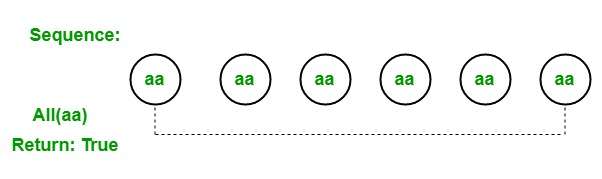Related Articles

# LINQ | Quantifier Operator | All

• Last Updated : 26 May, 2019

In LINQ, quantifier operators are used to returning a boolean value which shows that whether some or all elements satisfies the given condition. The Standard Query Operator supports 3 different types of quantifier operators:

1. All
2. Any
3. Contains

#### All Operator

The All operator is used to check each and every element in the sequence if all the elements satisfy the given condition then it will return true. Otherwise, return false. For example, we have a sequence, i.e, aa, aa, aa, aa. To check if all the elements of the sequence are “aa” we use All operator, this operator checks each and every element present in the given sequence and return true because all the elements present in the given sequence satisfy the given condition.Important Points:

• It does not support query syntax in C# and VB.Net languages.
• It support method syntax in both C# and VB.Net languages.
• It present in both the Queryable and Enumerable class.
• It will throw ArgumentNullException if the given source or the given condition is null.
• It does not return value instead of this it return true or false.
• The return type of this operator is System.Boolean.

Example 1:

 `// C# program to illustrate the``// use of All operator``using` `System;``using` `System.Linq;``using` `System.Collections.Generic;`` ` `class` `GFG {`` ` `    ``static` `public` `void` `Main()``    ``{`` ` `        ``// Data source``        ``int``[] sequence1 = {112, 44, 55, 66,``                              ``77, 777, 56};``                               ` `        ``string``[] sequence2 = {``"aa"``, ``"aa"``, ``"aa"``,``                             ``"aa"``, ``"aa"``, ``"aa"``};`` ` `        ``// Check the sequence1 contain``        ``// all element as 77``        ``// Using All operator``        ``var` `result1 = sequence1.All(seq => seq == 77);`` ` `        ``Console.WriteLine(``"Is the given sequence contain"``+``                     ``" all element as 77 : {0}"``, result1);`` ` `        ``// Check the sequence2 contain``        ``// all element as aa``        ``// Using All operator``        ``var` `result2 = sequence2.All(seq => seq == ``"aa"``);`` ` `        ``Console.WriteLine(``"Is the given sequence contain"``+``                   ``" all element as 'aa' : {0}"``, result2);``    ``}``}`
Output:
```Is the given sequence contain all element as 77 : False
Is the given sequence contain all element as 'aa' : True
```

Example 2:

 `// C# program to check in the employee present``// in the company are all female employee``using` `System;``using` `System.Linq;``using` `System.Collections.Generic;`` ` `// Employee details``public` `class` `Employee {`` ` `    ``public` `int` `emp_id``    ``{``        ``get``;``        ``set``;``    ``}`` ` `    ``public` `string` `emp_name``    ``{``        ``get``;``        ``set``;``    ``}`` ` `    ``public` `string` `emp_gender``    ``{``        ``get``;``        ``set``;``    ``}`` ` `    ``public` `string` `emp_hire_date``    ``{``        ``get``;``        ``set``;``    ``}`` ` `    ``public` `int` `emp_salary``    ``{``        ``get``;``        ``set``;``    ``}``}`` ` `class` `GFG {`` ` `    ``// Main method``    ``static` `public` `void` `Main()``    ``{``        ``List emp = ``new` `List() {`` ` `            ``new` `Employee() {emp_id = 209, emp_name = ``"Anjita"``, emp_gender = ``"Female"``,``                                    ``emp_hire_date = ``"12/3/2017"``, emp_salary = 20000},`` ` `            ``new` `Employee() {emp_id = 210, emp_name = ``"Soniya"``, emp_gender = ``"Female"``,``                                    ``emp_hire_date = ``"22/4/2018"``, emp_salary = 30000},`` ` `            ``new` `Employee() {emp_id = 211, emp_name = ``"Rohit"``, emp_gender = ``"Male"``,``                                  ``emp_hire_date = ``"3/5/2016"``, emp_salary = 40000},`` ` `            ``new` `Employee() {emp_id = 212, emp_name = ``"Supriya"``, emp_gender = ``"Female"``,``                                      ``emp_hire_date = ``"4/8/2017"``, emp_salary = 40000},`` ` `            ``new` `Employee() {emp_id = 213, emp_name = ``"Anil"``, emp_gender = ``"Male"``,``                                ``emp_hire_date = ``"12/1/2016"``, emp_salary = 40000},`` ` `            ``new` `Employee() {emp_id = 214, emp_name = ``"Anju"``, emp_gender = ``"Female"``,``                                  ``emp_hire_date = ``"17/6/2015"``, emp_salary = 50000},``        ``};`` ` `        ``// Query to check in the employee present``        ``// in the company are all female employee``        ``// Using All method``        ``var` `res = emp.All(e => e.emp_gender == ``"Female"``);``         ` `        ``Console.WriteLine(``"Is all the employees are female?: {0}"``, res);``    ``}``}`
Output:
```Is all the employees are female?: False
```

My Personal Notes arrow_drop_up